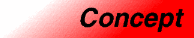# ForwardContainerCategory: containers Component type: concept

## Description

A Forward Container is a Container whose elements are arranged in a definite order: the ordering will not change spontaneously from iteration to iteration. The requirement of a definite ordering allows the definition of element-by-element equality (if the container's element type is EqualityComparable) and of lexicographical ordering (if the container's element type is LessThanComparable).

Iterators into a Forward Container satisfy the ForwardIterator requirements; consequently, Forward Containers support multipass algorithms and allow multiple iterators into the same container to be active at the same time.

## Associated types

No additional types beyond those defined in Container. However, the requirements for the iterator type are strengthened: the iterator type must be a model of ForwardIterator.

## Notation

 `X` A type that is a model of Forward Container `a`, `b` Object of type `X` `T` The value type of `X`

## Valid expressions

In addition to the expressions defined in Container, EqualityComparable, and LessThanComparable, the following expressions must be valid.

Name Expression Type requirements Return type
Equality `a == b` `T` is EqualityComparable Convertible to `bool`
Inequality `a != b` `T` is EqualityComparable Convertible to `bool`
Less `a < b` `T` is LessThanComparable Convertible to `bool`
Greater `a > b` `T` is LessThanComparable Convertible to `bool`
Less or equal `a <= b` `T` is LessThanComparable Convertible to `bool`
Greater or equal `a >= b` `T` is LessThanComparable Convertible to `bool`

## Expression semantics

Semantics of an expression is defined only where it is not defined in Container, EqualityComparable, or LessThanComparable, or where there is additional information.

Name Expression Precondition Semantics Postcondition
Equality `a == b`   Returns `true` if `a.size() == b.size()` and if each element of `a` compares equal to the corresponding element of `b`. Otherwise returns `false`.
Less `a < b`   Equivalent to `lexicographical_compare(a,b)`

## Complexity guarantees

The equality and inequality operations are linear in the container's size.

## Invariants

 Ordering Two different iterations through a forward container will access its elements in the same order, providing that there have been no intervening mutative operations.